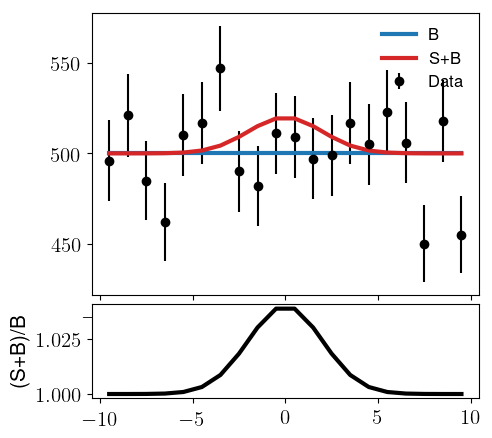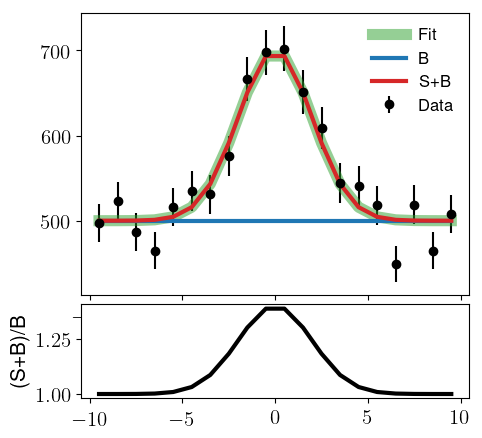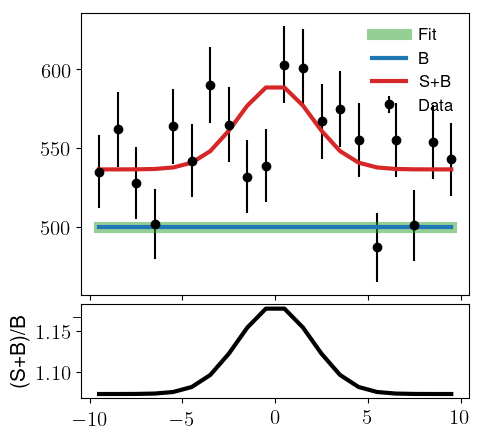# RooRealSumPdf in AsymptoticCalculator calculation

Hi, I would like to do a simple CLs comparison between a B-only model and S+B model. Since in the full version the S model is negative, I want to use RooRealSumPdf to add the S and B shapes:

``````model = RooRealSumPdf("model","model",
RooArgList(bkg, sig),
RooArgList(nBkgExp,nSig)
)
``````The problem is when I configure the B and S+B `ModelConfig`s and put these into the `AsymptoticCalculator`, I am unable to call `GetHypoTest()`. (Oddly enough, this seems to work when I use `RooAddPdf` instead of `RooRealSumPdf`.) The error message is:

``````[#0] PROGRESS:Eval -- AsymptoticCalculator: Building Asimov data Set
[#0] ERROR:InputArguments -- A counting model pdf must be either a RooProdPdf or a RooPoisson or a RooGaussian
[#0] ERROR:InputArguments -- AsymptoticCalculator: Error : Asimov data set could not be generated
[#0] PROGRESS:Eval -- AsymptoticCalculator: Building Asimov data Set
[#0] ERROR:InputArguments -- A counting model pdf must be either a RooProdPdf or a RooPoisson or a RooGaussian
[#0] ERROR:InputArguments -- AsymptoticCalculator: Error : Asimov data set could not be generated
[#0] ERROR:InputArguments -- AsymptoticCalculator::GetHypoTest - Error initializing Asymptotic calculator - return NULL result
``````

I have attached a full stand-alone example of this (See line 58. errorDemoAsymptoticCalculator.py (5.1 KB)). I must be misunderstanding something about how AsymptoticCalculator works, but I’m not sure what. Is there something I’m missing? Can I use AsymptoticCalculator to set this kind of limit?

Hello @aaronsw,

the Asymptotic calculator indeed only supports PDFs where you can count the number of events, and additionally a Gaussian distribution. You can make each PDF countable by extending it, i.e., lifting the “normalised to one” condition.
The reason that it works with the RooAddPdf is that it automatically switches to an extended mode if you have as many PDFs as coefficients, because in this case the sum of all coefficients is the expected number of events.

The RooRealSumPdf can be extended in the same way, but it requires an active decision to extend it. See:
https://root.cern.ch/doc/master/classRooRealSumPdf.html
and switch the last (default) argument of the constructor to `True`:

``````    model = RooRealSumPdf("model","model",
RooArgList(bkg, sig),
RooArgList(nBkgExp,nSig),
True #This is important!
)
``````

And check the value of:

``````model.canBeExtended()
``````

to check whether the extension was successful.

Hi @StephanH,

Thanks very much. I made this change, but it seems that that the model wants to make nSig very negative. For example I did fits with this:

Using RooAddPdf (green line fit to data)Using RooRealSumPdf (green line fails to fit the data, and will go negative if allowed)When I call `GetHypoTest()` I only get `nan` values. `model.canBeExtended()` is True.

Is there something else I need to do? Thanks very much for the help.
Aaron

As a follow up: the fit works for `RooRealSumPdf` if I remove the `AsymptoticCalculator(data, h0_model, h1_model)` line. So is something happening inside `AsymptoticCalculator` that modifies the PDF?

I think this may be a separate issue, so I made another post here: Difference between RooRealSumPdf and RooAddPdf

The particular confusion with RooRealSum in AsymptoticCalculator has been resolved, thanks @StephanH

This topic was automatically closed 14 days after the last reply. New replies are no longer allowed.